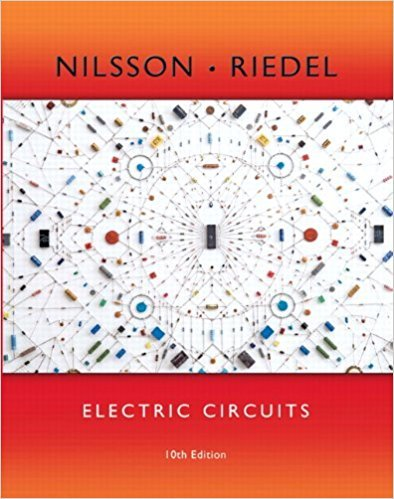×
×

# a) Write a set of mesh-current equations for the circuit in Example 6.6 if the dot onISBN: 9780133760033 390

## Solution for problem 6.6 Chapter 6.4

Electric Circuits | 10th Edition

• Textbook Solutions
• 2901 Step-by-step solutions solved by professors and subject experts
• Get 24/7 help from StudySoup virtual teaching assistantsElectric Circuits | 10th Edition

4 5 1 385 Reviews
14
4
Problem 6.6

a) Write a set of mesh-current equations for the circuit in Example 6.6 if the dot on the 4 H inductor is at the right-hand terminal, the reference direction of is reversed, and the resistor is increased to b) Verify that if there is no energy stored in the circuit at and if the solutions to the differential equations derived in (a) of this Assessment i2 = -0.01 - 0.99e-4t + e-5t A. i1 = -0.4 - 11.6e-4t + 12e-5t A, ig = 1.96 - 1.96e-4t t = 0, A, 60 780 . ig Answer: (a) and (b) verification. -16(dig = >dt); 20i1 + 16(di2>dt) + 800i 8(di1 - 2 >dt) -5ig - 8(dig = >dt) 4(di1>dt) + 25i1 + 8(di2>dt) - 20i2

Step-by-Step Solution:
Step 1 of 3

Storage and Databases Notes 10­18­16 Exam #2 A. Input­ process of adding data content to database tables. a. Data has important attributes i. Data type (numbers, letters) ii. Sometimes it’s required. B. Data Validation a. Data Base Management Systems (DBMS)­ provides users and programmers with a systematic...

Step 2 of 3

Step 3 of 3

##### ISBN: 9780133760033

Unlock Textbook Solution